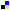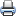# WDM Network DesignSaveDiggDel.icio.usPrint

### Chapter Description

This sample chapter explores some of the design constraints involved in the WDM network design.

## Effect of Chromatic Dispersion on Transmission Length and Induced Power Penalty

Group velocity dispersion (chromatic dispersion) is a primary cause of concern in high bit rate (> 2.5 Gbps) single-mode WDM systems. As explained previously, dispersion in an optical pulse creates pulse broadening such that the pulse spreads into the slots (in the time domain) of the other pulses. This not only causes ISI, but it also introduces a power penalty, which can cause degradation of the system's SNR. As shown in the next section, OSNR is a true figure of merit for optical communications. The power penalty due to dispersion is shown in Equation 4-7.

#### Equation 4-7In the preceding equation, λ is the spectral width and σ0 is the pulse width. Further for SMF fibers, dispersion parameter D = 17 ps/Km – nm. The limit on transmission distance is shown as in Equation 4-8.

#### Equation 4-8Therefore, the following equation is true.K is a constant; therefore, as B increases, L decreases with the square root of B.

4. Design of a Point-to-Point Link Based on Q-Factor and OSNR | Next Section Previous Section